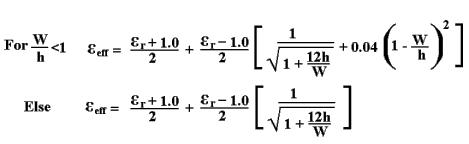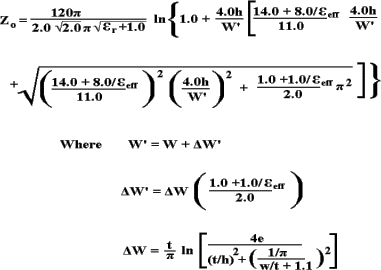# Microstrip Transmission Line Characteristic Impedance Calculator Using a Formula by Brian C. Wadell

The characteristic impedance (Zo) of a microstrip transmission line can be calculated using the active calculator or the formulas at the bottom of the page.Dielectric Constant (εr): Track Width (W): mm Track Thickness (t): mm Effective Dielectric Constant (εeff): Dielectric Thickness (h): mm Characteristic Impedance (Zo): Ohms

This calculator uses Visual Basic and will function in Internet Explorer and other browsers with Visual Basic plug-ins. For more information see About our calculators

The following formulas were derived from formulas used in an article by Rick Hartley http://www.jlab.org/accel/eecad/pdf/050rfdesign.pdf  It is not too clear from the article by Rick Hartley where to use εr and where to use εeff (although there is a note to use eeffinstead of er). The substitutions selected give results that are almost identical to the "benchmarks" in http://www.polarinstruments.com/support/cits/IPC1999.pdfand these benchmarks were obtained by numerical methods. The accuracy of results obtained from this calculator can be checked by making a sample PCB and measuring the resulting impedance. Guidance for this can be found at Measuring Characteristic Impedance of PCB tracks using a Vector Network AnalyserWhere εr is the Relative Dielectric Constant

W is the Width of track

t is the Thickness of track (1 oz/ft2 = 0.035 mm)

h is the Thickness of dielectric

The above formulas are also in Transmission Line Design Handbook by Brian C. Wadell, Artech House 1991, with some differences in calculating εeff. The main formula is attributable to Harold A. Wheeler and was published in, "Transmission-line properties of a strip on a dielectric sheet on a plane", IEEE Tran. Microwave Theory Tech., vol. MTT-25, pp. 631-647, Aug. 1977. The effective dielectric constant formula is from: M. V. Schneider, "Microstrip lines for microwave integrated circuits," Bell Syst Tech. J., vol. 48, pp. 1422-1444, 1969.

This calculator is provided free by Chemandy Electronics in order to promote the FLEXI-BOX